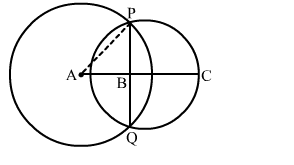# In the given figure, A and B are the centres of two circles having radii 5 cm and 3 cm respectively and intersecting at points P and Q respectively.

Question:

In the given figure, A and B are the centres of two circles having radii 5 cm and 3 cm respectively and intersecting at points P and Q respectively. If AB = 4 cm, then the length of common chord PQ is
(a) 3 cm
(b) 6 cm
(c) 7.5 cm
(d) 9 cmSolution:

(b) 6 cm
We know that the line joining their centres is the perpendicular bisector of the common chord.
Join AP.
Then AP = 5 cm; AB = 4 cm
Also, AP2 = BP2 + AB2
Or BP2  = AP2 - AB2
Or BP2  = 52 - 42
Or BP = 3 cm
∴ ΔABP is a right angled and PQ = 2 × BP = (2 × 3) cm = 6 cm